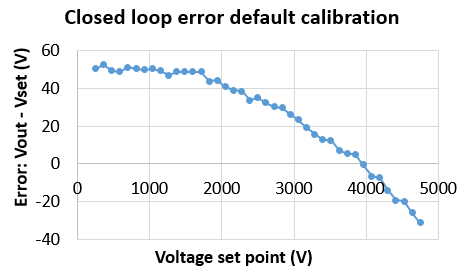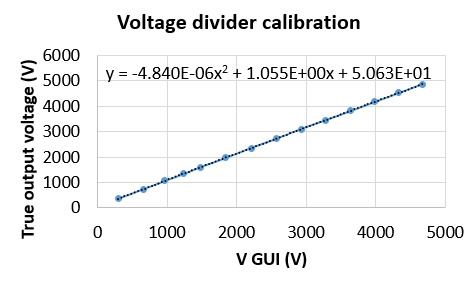# Default and manual calibration

There are basically 3 options to calibrate the HVPS depending on the material that you have at your disposal. Using the default calibration (section 1) requires no tool but is the less accurate. You can perform a manual calibration (section 2) if you have a high voltage voltage probe and a multi-meter. The precision of this method depends on the number of point you take. Finally, if you have a NI-DAQ unit, you can perform an automatic calibration of the HVPS.

# 1 Default calibration

When you perform the initial configuration described in section 1.2 above, a default calibration factor is stored into the HVPS. The HVPS ADC transforms a 0-5V analogue voltage to a 10 bit values. It therefore expects to map 0 to 5V between 0V and the maximal rating of the HVPS. However, the resistive divider which steps down the high voltage to a value that can be fed to the microcontroller has a slightly higher ratio to enable the detection of an overvoltage. The ratio for the different HVPS voltage ratings is given on this page in column c1. When you perform the configuration mentioned above, the HVPS is initialized with a linear correction factor c1 given in the column c1 of the table.

This default configuration is a good guess, but is not extremely precise, due to the uncertainty on the resistance values and other imperfections. The graph below shows the error in volt between the set point and the true output voltage for a 5kV HVPS. It can be seen that the error is (for this particular unit) comprised between +/- 50 V, i.e. 1% of the full scale## 2 Manual Calibration

It is recommended to calibrate each HVPS to increase the precision. We recommend to use the calibration routine included with the interface, but this requires some external equipment and components. If you don’t have them, you can proceed with a manual calibration. The only equipment you need is a high voltage multimeter probe that can sustain the full voltage output of your HVPS (such as the High-voltage probe, 34136A, Keysight). Although these multimeter probes have a defined voltage division ratio (typically 1000), a better accuracy can be obtained by performing a calibration of the probe (see section 2 of the automatic calibration page)

Here is how to proceed to perform a manual calibration:

1. Establish a direct serial connection to the HVPS
2. Set the calibration factors C0=0, C1=1, and C2=0 by issuing the commands
SC0 0
SC1 1
SC2 0
It is important to disable any correction before proceeding to the next step. setting C0 and C2 to 0 and C1 to 1 leads to no correction at all: y=c1 x = x.
3. Connect the output of the SHVPS to the HV probe and the multimeter
4. Launch the GUI
5. Place the HV enable switch on position 1
6. Select different voltages using the voltage set point dial. Write down the voltage read by the HVPS (feedback voltage in the GUI), and the voltage indicated by your multimeter.
7. Multiply the voltage read on the multimeter by the probe ratio (e.g. 1000), or by your own calibration equation to obtain the true output voltage
8. On a graph, plot the true output voltage form the previous step (y axis), as a function of the voltage read by the HVPS (x axis).
9. Use curve fitting to obtain the correction coefficients. In a perfect linear world, we physically expect to obtain a curve of the form y=c1 x. However, we have found that a correction equation of the form y=c0 + c1 x + c2 x^2 leads to a much better accuracy. This 2nd order polynomial correction is implemented in the HVPS with the coefficient c0, c1, and c2. But you can easily used a purely linear correction if you prefer by assigning a value of 0 to c0 and c2.
10. Once you have calculated the coefficients for your HVPS, close the GUI and establish a direct serial connection to the HVPS.
11. Set the coefficients to the values you desire using the commands
SC0 xx.xxx
SC1 xx.xxx
SC2 xx.xxx
where xx.xxx is the value you want to assign to each correction coefficient. Important: there is a 1E-6 multiplier for coefficient c2: if you calculate a coefficient c2 of 1.45e-6, you have to enter the command SC2 1.45

## 2.1 ExampleThe graph above show a manual calibration of a 5kV HVPS with 14 points. The voltage read by the HVPS and displayed in the interface is on the x axis, and the voltage read by the voltmeter (after correction by the probe calibration) is on the y axis. A 2nd order polynomial is fitted to the data, leading to the equation displayed on the graph. To enter this calibration into the HVPS, the following commands needs to be used:

• SC0 50.63
• SC1 1.055
• SC2 -4.84